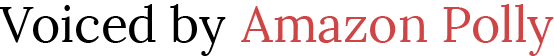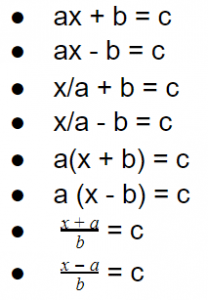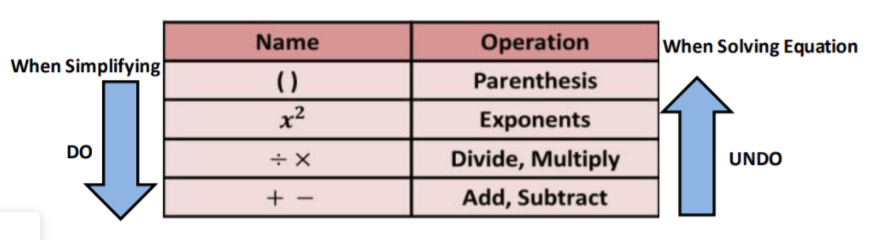# Solving Two-Step Equations

 Would You Rather Listen to the Lesson?Once they’re fluent in solving one-step equations, algebra 1 students can dive into solving two-step equations. This can sometimes be a tricky process, as it’s easy to mess up the order of operations, that is, the order of undoing operations to solve equations.

So if you’re a math teacher struggling with this topic – know that you’re not alone! There are several math guidelines that you can apply to ensure that teaching children to solve two-step equations is nothing but smooth sailing!

## What Is Solving Two-Step Equations?

Remind them that solving an equation refers to the process of providing a number that can be plugged in for the variable so that we end up with a true statement. Explain that one-step equations are called that because we solve them in one step.

In other words, we perform only one operation, such as addition, subtraction, multiplication, or division, to isolate the variable and thus solve the equation. Provide an example of a one-step equation, such as 3 + x = 12, x – 3 = 7, x + 4 = -10 etc.

Now that everyone is clear on the steps needed to solve a one-step equation, you can simply define a two-step equation as an algebraic expression that requires two steps to be solved. That is, two-step equations require performing two separate operations to isolate the variable.

## How to Solve Two-Step Equations

### Review Properties of Equality

Solving equalities requires knowledge of the properties of equality, as we need to apply one or several of these properties while solving the given equation. Remind children of the properties of equality, including:

• the reflexive property
• the transitive property
• the subtraction property
• the multiplication property
• the division property
• the substitution property

You may also want to check out this article for further explanation on the properties of equality.### Review One-Step Equations

Solving two-step equations is similar to solving one-step equations, with the difference being that there are two steps. So it makes sense to review one-step equations first. Remind children how we solve one-step equations. For instance, find x in:

x + 18 = 21

To isolate x, we need to do the inverse of that is done to the variable (x), that is, undo the operation. As the inverse of adding 18 is subtracting 18, we’ll subtract 18 and must do so on both sides. So we’ll only perform one step – subtraction. By doing so, we’ll get:

x + 18 – 18 = 21 – 18

x = 3

So there it is – the solution to our one-step equation is 3! Just make sure to highlight to students that whatever we do to one side, we must perform the same thing on the other side of the equation.

You can also use our free Activity Worksheet on one-step equations. Simply print out copies for all students and let them play the game to refresh their knowledge of one-step equations. It may also be useful to check out this article as an introduction to equations.

### Two-Step Equations

#### Forms of Two-Step Equations

Introduce the different forms that two-step equations can take. If the variable in the given equation is x, this includes the following forms:#### Solving Two-Step Equations: Example 1

Write an example of a simple two-step equation on the whiteboard, for instance, 3x + 2 = 14

Point out that this equation is of the form ax + b = c. That means there are two operations involved, multiplication (ax) and addition (ax + b) that we need to undo in order to solve the equation.

Now that we’ve identified the two steps that we do perform, in which order do we perform these operations?

Students are already familiar with the order of operations in math, that is, first we do operations inside the parenthesis, then exponents, then multiplication and division, and finally, addition and subtraction.

Remind students that solving equations requires undoing any operations that the variable is involved in so that we manage to isolate it. So that means that we’ll apply the order of operations in reverse. You can draw a chart like this so that it’s easier for students to remember the way we undo these operations:Explain that for our equation, this means that we’ll first undo addition and then we’ll undo multiplication. So in step 1, to undo addition, we’ll simply add – 2 on both sides:

3x + 2 = 14

3x + 2 – 2 = 14 – 2

3x = 12

In step 2, to undo multiplication, we’ll divide by three on both sides to be able to find the variable:

3x = 12

x/3 = 12/3

x = 4

#### Solving Two-Step Equations: Example 2

When teaching how to solve two-step equations, it’s also useful to provide at least one example of an equation that contains parenthesis, as they may be confusing. Normally, students know that in math we need to first perform the operation inside the parenthesis.

However, according to the above chart, when we’re solving equations and undoing operations, we need to leave the parenthesis for the very end. So we do them last of all. Write an example on the whiteboard of a two-step equation that contains parenthesis, such as:

2 (x + 2) = 8

Explain to students that we’ll first identify the steps we need to perform. By following the rules in the chart, we observe that the first operation that we need to undo is multiplication. To undo it, we’ll divide both sides of the equation by two.After performing the division on both sides, we’ll get:

(x + 2) = 4

Explain that since there’s nothing else next to the group in the parenthesis anymore, we don’t even need the parenthesis and can simply delete it. That is, we can simply write:

x + 2 = 4

Point out that now we can move on to step 2, that is, undoing addition. To achieve this, we’ll subtract 2 on both sides of the equation:

x + 2 – 2 = 4 – 2

x = 2

You can also decide to include a video lesson instead of just teaching how to solve two-step equations on a whiteboard. This free video by Khan Academy contains a simple introduction to solving two-step equations. In addition, this free video contains simple instructions on how to solve two-step equations, including equations with parenthesis.

## Activities to Practice Solving Two-Step Equations### Maze Activity

As a teacher or a homeschooling parent, you may want to find ways to practice solving equations without losing children to boredom! So why not give it a try with a fun game, such as using a maze activity?

To implement this activity in your classroom, make sure to print out a sufficient number of copies from our free Maze Activity Worksheet. The worksheet contains all sorts of two-step equations, including equations with parentheses and without parentheses.

Divide students into pairs and hand out one copy of the worksheet per student. Explain the rules of the game. The first student to reach the end of the maze wins the game!

If you’re a homeschooling parent, you can easily adapt the maze activity to an individual game where your child tries to finish all equations by being timed!

## Before You Leave…

If you liked the guidelines in this article, we have a whole lesson that is dedicated to this topic!

So if you’re looking for more math materials for children of all ages, sign up for our emails for plenty of free content! Feel free to also head over to our blog.

And if you’d like to become a member, sign up at Math Teacher Coach!